# Help on Formula: if function

Hi all,

Please need your help. I'm new to formulas and I've been trying to figure out the right formula for my project status and progress report but I kept getting errors. I have tried the below formula in excel and it worked but not in smartsheet (the smartsheet formula below is another trial I did that did not work).

Excel: =IF(H3>=F3,"Completed",IF(AND(G3=" ",H3=" "),"Active","Not Due"))

Smartsheet: =IF(AND([Actual End Date]1 = >[Planned End Date]1, "Completed", IF(ISBLANK([Actual End Date]1), "Active", IF([Actual Start Date]1 < [Planned Start Date]1, "Not Due"))))

What I would like to determine in this report is the Status of the entire project based on the subtasks. So if any of the children have an actual end date then the project is Complete but if any of the children have blanks on the actual start date then it should be marked as Not Started and Active if the actual start date is filled but should be equal to the current date.

The Progress column should be determined based on the planned dates. So if the actual end date is less than or equal to the planned end date, then it should be marked as On-time. Delayed if the actual end date is greater than the planned end date and Not Due if planned start date is in the future so actual start date cell must be blank.

Many thanks in advance for the support,

Grace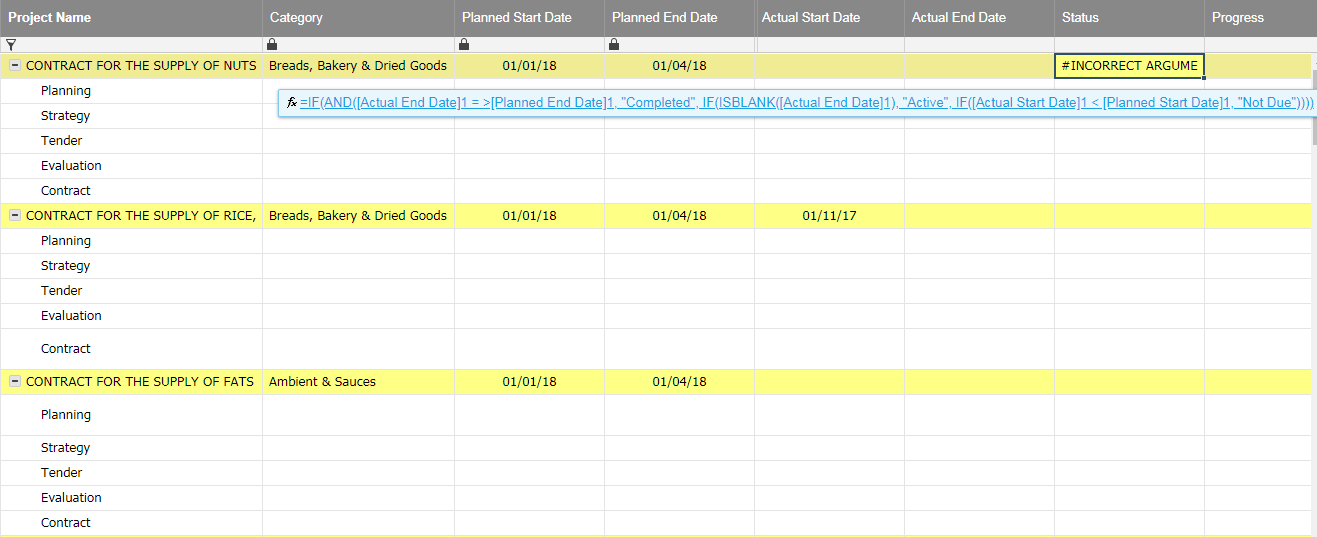«1

• Hi Grace,

You shouldn't place an = sign before a > (as it will error) and it looks like you've got a stray AND in there, as you hadn't closes it before trying to specify the True & False values for your IF statement.

=IF([Actual End Date]1 > [Planned End Date]1, "Completed", IF(ISBLANK([Actual End Date]1), "Active", IF([Actual Start Date]1 < [Planned Start Date]1, "Not Due")))

Hope this is what you were after.

Kind regards,

Chris McKay

• Hello Grace,

Thanks for the question. In looking this over, I'm not sure I completely understand what you're wanting the formula to do. In looking at the two formulas you gave as examples, these look like they would be for the Status column on each row possibly due to the results of "Complete" and "Active", however they also include the result "Not Due" which you explain later you want for the Progress column.

The Excel formula you provided should work within Smartsheet in the same way as it does in Excel, as long as the cell references are updated to look at the correct cell names. Both Excel and Smartsheet reference cells by using the column name followed by the row number, however Excel uses letters for their column names and Smartsheet uses the names you give to a column.

Assuming this is how the columns align between the two:

• F = Planned End Date
• G = Actual Start Date
• H = Actual End Date

This is how your Excel formula would look:

=IF([Actual End Date]3 >= [Planned End Date]3, "Completed", IF(AND([Actual Start Date]3 = " ", [Actual End Date]3 = " "), "Active", "Not Due"))

As for the Smartsheet formula you provided, there's a few syntax related issues that are causing the error you're seeing. Here's this formula with those things resolved:

=IF([Actual End Date]1 >= [Planned End Date]1, "Completed", IF(ISBLANK([Actual End Date]1), "Active", IF([Actual Start Date]1 < [Planned Start Date]1, "Not Due")))

When using the >, <, and = operators together within arguments, the order they're in matters. This for instance => will result in an error, where this >= will not. More on this can be found in the "Formula Operators" section of this help center article (https://help.smartsheet.com/articles/2476171). The only other thing was the AND function at the front of the formula wasn't closed in the correct spot and technically wasn't needed, so I removed it and the parentheses at the end.

That being said, these formula still may not do what you'd like for them to. From the sounds of it you'd like for the Status column of each row to be set to either Not Started, Active, or Complete, when certain conditions are met, and the Progress column for each row set to either On-time, Delayed, or Not Due, when certain other conditions are met.

From your description, it's hard to tell exactly which conditions apply to which status or progress or if this is really what you're looking for. You also mention being able to see the status and progress for the entire project. Are you instead looking for the status and progress to only show in the parent rows?

Either way, more information would be needed in order to help build out a formula. I'm happy to help further if you can lay out exactly what you'd looking to have these formulas do. Laying out the conditions and results in a bulleted list suck as this may help as well:

• Not Started - Actual Start Date for the row is blank
• Active - Actual Start Date for the row is todays date or in the past
• Complete - Actual End Date for the row has a date
• Hi Chris and Robert,

Thank you both for the response and apologies for the confusion. I am looking for the status and progress of all rows, not just the parent rows. And yes I agree to list all the information in bullets to help build out the formulas. I will list down the texts required for each column first and then its conditions.

The texts required for the columns Status and Progress:

Status Column

• Active
• Complete
• Not started

Progress Column

• Delayed
• On-time
• Not due

I have filled in some dummy dates on one project to give an example of what I want to achieve on this report (see image below).

And here are the conditions:

Status Column

• Active - yes, the Actual Start Date for the row should be today's date or in the past
• Complete - yes, the Actual End Date for the row has a date regardless if it is in the present
• Not started - yes, Actual Start Date for the row is blank

Progress Column

Now here's the complicated part and where I am not sure if possible to put in one formula as I am seeing 2 possible conditions (or maybe more that I haven't realised yet) for each.

• Delayed
1. If the status of the row is Active or Complete, then the Progress for the row should be marked as Delayed if the Actual End Date is greater than or not equal to the Planned End Date, blank (Active) or not blank (Complete)
2. If the status of the row is Not started, then the Progress for the row should be marked as Delayed if the Actual Start Date is blank
• On-time
1. If the status of the row is Active and the Planned Start Date is less than or equal to the Actual Start Date, then it should be marked as On-time
2. If the status of the row is Complete and the Planned End Date is less than or equal to the Actual End Date, then it should be marked as On-time
• Not due
1. If the status of the row is Not started and the Planned Start Date is in the future, then it should be marked as Not due
2. If the status of the row is Active and the Planned End Date is in the future, then it should be marked as Not due

I hope I have covered everything that's needed but let me know if anything is unclear.

Best,

Grace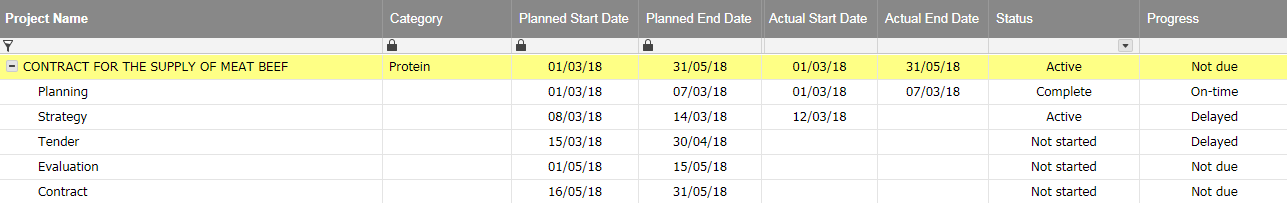• Hi Grace,

Thanks for the clarification on the status column. Here's a formula that should work based on what you've mentioned:

=IF(ISDATE([Actual End Date]@row), "Complete", IF(ISBLANK([Actual Start Date]@row), "Not Started", IF([Actual Start Date]@row <= TODAY(), "Active")))

For the progress column however, there are a few contradictions I'm seeing and some things that are still unclear. Since the status column is being set automatically based on the dates, and the progress is also being set on the dates, the status shouldn't make a difference when it comes to the progress. Can you explain the progress needs without the status involved, similar to how you did with the status explanation?

• Hi Robert,

Thanks for the status formula. It worked perfectly!As for the Progress column, sure, I'll try to simplify it.

Progress Column

• Delayed
1. If the Actual Start Date for the row is greater than or not equal to the Planned Start Date
2. If the Actual End Date for the row is greater than or not equal to the Planned End Date
• On-time
1. If the Planned Start Date for the row is less than or equal to the Actual Start Date
2. If the Planned End Date for the row is less than or equal to the Actual End Date
• Not due
1. If the Planned Start Date for the row is in the future
2. If the Planned End Date for the row is in the future

Now it did looked a lot less complicated than my previous comment. However, putting it without the status makes it quite a bit confusing to me now. I'm afraid I might have missed out some points but I hope it makes more sense to you to build the formulas.

It's quite difficult to put explanations in writing so please do let me know if anything is unclear.

Best,

Grace

• Hello Grace,

There's still some contradictions such as "the Actual Start Date for the row is greater than the Planned Start date" is the same thing as "the Planned Start Date for the row is less than the Actual Start Date", but they're listed as having different results. However, I've created the following formula with what I think you're looking for:

=IF(OR([Planned Start Date]@row > TODAY(), [Planned End Date]@row > TODAY()), "Not Due", IF(OR([Actual Start Date]@row > [Planned Start Date]@row, [Actual End Date]@row > [Planned End Date]@row), "Delayed", IF(OR([Actual Start Date]@row <= [Planned Start Date]@row, [Actual End Date]@row <= [Planned End Date]@row), "On-time")))

Try this out and see if it does what you're looking for. I'm happy to help further if you have any further questions or needs on this.

• Hi Robert,

I tested the above formula however it's giving a different answer (see image Test 1) so I tried to switch "Delayed" and "On-time" (see results on image Test 2). It did work however the logic does not look quiet right.

=IF(OR([Planned Start Date]@row > TODAY(), [Planned End Date]@row > TODAY()), "Not Due", IF(OR([Actual Start Date]@row > [Planned Start Date]@row, [Actual End Date]@row > [Planned End Date]@row), "On-time", IF(OR([Actual Start Date]@row <= [Planned Start Date]@row, [Actual End Date]@row <= [Planned End Date]@row), "Delayed")))

On image Test 3, I added an equal sign around the "On-time" part.

=IF(OR([Planned Start Date]@row > TODAY(), [Planned End Date]@row > TODAY()), "Not Due", IF(OR([Actual Start Date]@row >= [Planned Start Date]@row, [Actual End Date]@row >= [Planned End Date]@row), "On-time", IF(OR([Actual Start Date]@row <= [Planned Start Date]@row, [Actual End Date]@row <= [Planned End Date]@row), "Delayed")))

It gave a similar result but if the planned and start dates are blank it says On-time (on image 2 it says Delayed if the date columns are blank).

I am not really bothered if the blanks says something differently but if there's a way to make it in "Not Due" instead of On-time or Delayed would be better.

Also, what do you think about the switch that I did? Although I got the results that I am expecting, the formula's logic is incorrect.

Best,

Grace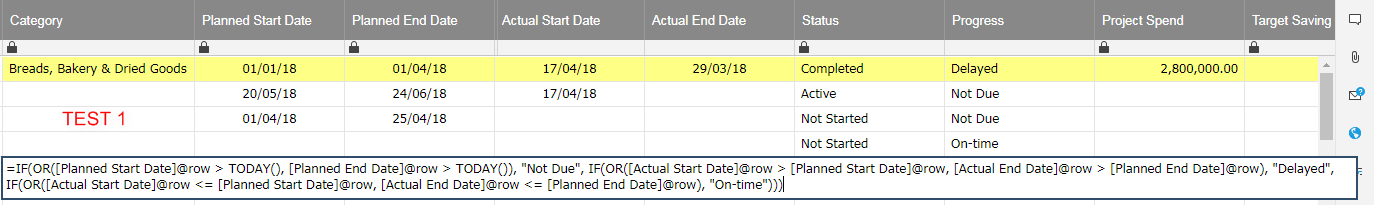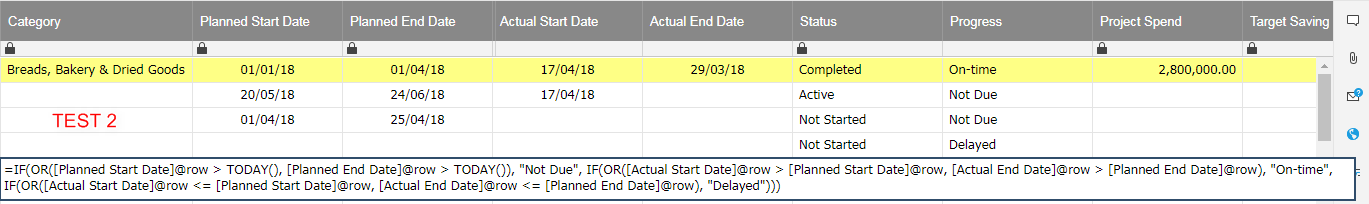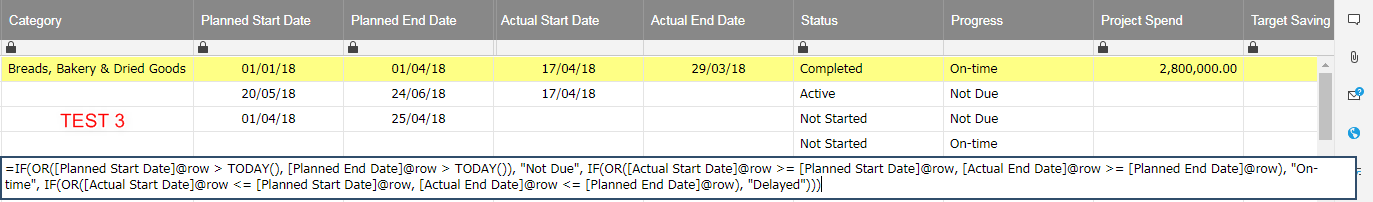• Hi Grace,

Formulas will find the first true statement from left to right and return that value. If one value should override another value, the order their put in the formula matters.

For instance, in the Test 2 image the first row shows as "On-time" because the formula first checks if either the "Planned Start Date" or "Planned End Date" is in the future, and then moves on and checks if either the "Actual Start Date" is greater than the "Planned Start Date" OR the "Actual End Date" is greater than the "Planned End Date". Since the "Actual Start Date" is greater than the "Planned Start Date", the formula stops here and returns "On-time" or how it was originally written it would return "Delayed.

From the sounds of it the formula I provided doesn't meet what you're looking for. I took another shot at it, this time just thinking about how I'd want it to work. This also keeps the cell blank if there's no "Planned Start Date" or "Planned End Date". Here's that formula:

=IF(OR(ISBLANK([Planned Start Date]@row), ISBLANK([Planned End Date]@row)), "", IF([Planned End Date]@row > TODAY(), IF([Planned Start Date]@row > TODAY(), "Not Due", IF(ISBLANK([Actual Start Date]@row), "Delayed", "On-time")), IF(AND(ISDATE([Actual End Date]@row), [Actual End Date]@row <= [Planned End Date]@row), "On-time", "Delayed")))

Try this out to see if it fits your needs better. If this returns any unwanted results for certain conditions, please let me know what these are as well as what is shown vs what you'd like.

• Hi Robert,

I can't thank you enough for helping me out on this. I tried the formula and it worked as expected for most of the lines. But here's another catch, my colleague and I were also arguing about this yesterday but both agreed in the end.

So here's what happened, to get a better understanding of this report we manually entered the Progress yesterday as how we understood it and surprisingly after testing the above formula, the same conditions appeared for the same lines we were arguing about.

On the image below notice the 2 projects in red, yes it is true that the projects are on-time based on the planned end dates. However, if you look at the planned start date vs the actual start date, the project actually started late and that's what we wish to reflect - "Active", "Delayed".

If the project owner was able to hit the deadline on or before the planned end date, then the expected Status and Progress would be "Completed", "On-time". The reason for this is to encourage the project owners to complete their projects on-time as they are being measured on a monthly basis.

As for the 2 lines with blue, yes it is true that the project is Not Due based on both planned start and end dates but we think that it is fairer for the project owners that the Progress would state as On-time since the project started earlier than expected which would then give an agreeable result on their performance review.

I hope I'm not too much of a pain but I do am learning a lot about if conditions with your help.

Kind regards,

Grace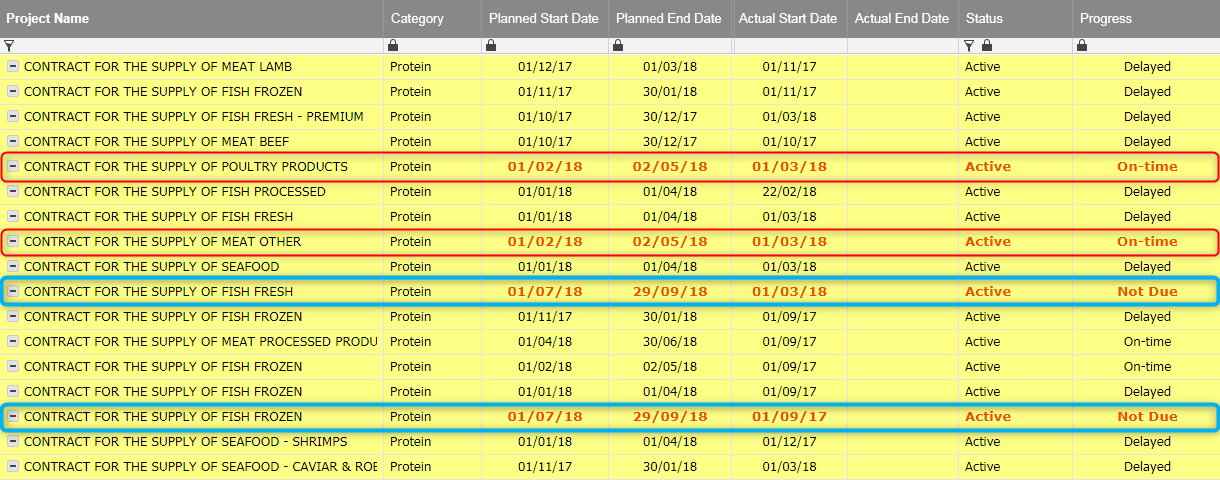• Hi Grace,

I'm happy to help, and glad that we're getting there. I've made a few revisions to the formula to account for those needs, and in testing it it seems like it's working as you're looking for now. Here's the formula:

=IF(OR(ISBLANK([Planned Start Date]@row), ISBLANK([Planned End Date]@row)), "", IF([Planned End Date]@row > TODAY(), IF([Planned Start Date]@row > TODAY(), IF(ISBLANK([Actual Start Date]@row), "Not Due", "On-time"), IF(OR(ISBLANK([Actual Start Date]@row), [Actual Start Date]@row > [Planned Start Date]@row), "Delayed", "On-time")), IF(AND(ISDATE([Actual End Date]@row), [Actual End Date]@row <= [Planned End Date]@row), "On-time", "Delayed")))

• Hi Robert,

Can we tweak it a little bit more, please? We're still getting Delayed status when the Actual Start Date is greater than Planned Start Date even if the Actual End Date is less than the Planned End Date.

The expected result is On-time if the project owner is able to complete it before the planned end date even if the project started late than planned.

Kind regards,

Grace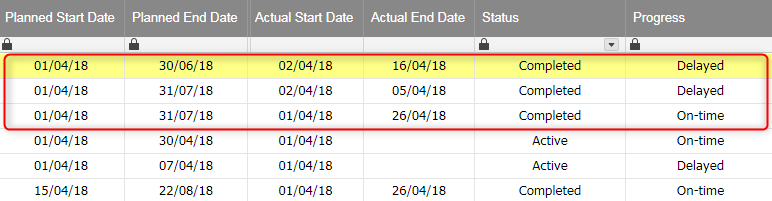• Hi Grace,

That makes sense to me, here's the updated formula for this:

=IF(OR(ISBLANK([Planned Start Date]@row), ISBLANK([Planned End Date]@row)), "", IF([Planned End Date]@row > TODAY(), IF([Planned Start Date]@row > TODAY(), IF(ISBLANK([Actual Start Date]@row), "Not Due", "On-time"), IF(OR(ISBLANK([Actual Start Date]@row), AND(ISBLANK([Actual End Date]@row), [Actual Start Date]@row > [Planned Start Date]@row)), "Delayed", "On-time")), IF(AND(ISDATE([Actual End Date]@row), [Actual End Date]@row <= [Planned End Date]@row), "On-time", "Delayed")))

• Hi Robert,

Unfortunately, the above did not work. I am also trying to adjust the formula but getting errors or the same results.

Kind regards,

Grace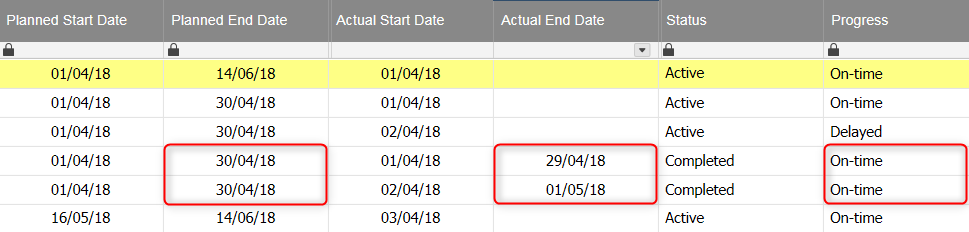• Hi Grace,

In thinking more and more about this, I've come up with a more simplistic formula for this that also resolves this latest issue. Here's the formula:

=IF(OR(ISBLANK([Planned Start Date]@row), ISBLANK([Planned End Date]@row)), "", IF(ISDATE([Actual End Date]@row), IF([Actual End Date]@row <= [Planned End Date]@row, "On-time", "Delayed"), IF(ISDATE([Actual Start Date]@row), IF(AND([Planned End Date]@row > TODAY(), [Actual Start Date]@row <= [Planned Start Date]@row), "On-time", "Delayed"), IF([Planned Start Date]@row > TODAY(), "Not Due", "Delayed"))))

This one should be a little easier to alter as well if other things need to be changed. I tried to make sure that it fits all of the previously mentioned scenarios, but please let me know if I missed anything.

• Hi Robert,

I am very happy to tell you that the formula finally worked! I had my presentation today with my boss and he was really happy about it too, all thanks to your help.Can't do it all if not because of this community. Thanks again for all the patience and support!

Best,

Grace

## Help Article Resources

Want to practice working with formulas directly in Smartsheet?

Check out the Formula Handbook template!